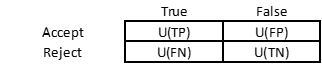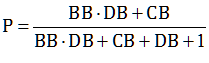The framework we used to describe a judge’s decision to convict or acquit a defendant based on the probability of Guilt can be generalised to any decision about whether to accept or reject a hypothesis. The utility function is defined over two states – True or False – and two decisions – Accept or Reject:The decision maker draws positive utility U(TP) from accepting a true hypothesis (True Positive) and negative utility U(FP) from accepting a false hypothesis (False Positive). And he draws positive utility U(TN) from rejecting a false hypothesis (True Negative) and negative utility U(FN) from rejecting a true hypothesis (False Negative). Based on these preferences, the threshold probability that leaves the decision maker indifferent between accepting and rejecting the hypothesis is:(1)

Hence:(2)

The decision maker accepts the hypothesis if he thinks the probability that the hypothesis is true is higher than P, and rejects it if he thinks it is lower. As in the judges’ case, we define BB=U(FP)/U(FN), CB=U(TN)/U(TP) and DB=-U(FN)/U(TP). BB is the ratio between the pain of a wrongful acceptance and the pain of a wrongful rejection. CB is the ratio between the pleasure of a rightful rejection and the pleasure of a rightful acceptance. And DB is the ratio between the pain of a wrongful rejection and the pleasure of a rightful acceptance. Using these definitions, (2) can be written as:(3)

which renders P independent of the utility function’s metric.

Again, with BB=CB=DB=1 we have P=50%: the hypothesis is accepted if it is more likely to be true than false. In most cases, however, the decision maker has some bias. We have seen a Blackstonian judge has BB>1: the pain of a wrongful conviction is higher than the pain of a wrongful acquittal. This increases the threshold probability above 50%. For example, with BB=10 we have P=85%: the judge wants to be at least 85% sure that the defendant is guilty before convicting him. On the other hand, the threshold probability of a ‘perverse’ Bismarckian judge, who dislikes wrongful acquittals more than wrongful convictions, is lower than 50%. For instance, with BB=0.1 we have P=35%: the judge convicts even if he is only 35% sure that the defendant is guilty.

In other cases, however, there is nothing perverse about BB<1. For instance, if the hypothesis is ‘There is a fire‘, a False Negative – missing a fire when there is one – is clearly worse than a False Positive – giving a False Alarm. This is generally the case in security screening, such as airport checks, malware detection and medical tests, where the mild nuisance of a False Alarm is definitely preferable to the serious pain of missing a weapon, a computer virus or a disease. Hence BB<1 and P<50%. As we have seen, with BB=0.1 we have P=35%. The same happens if CB<1: the pleasure of a True Positive – catching a terrorist, blocking a virus, diagnosing an illness – is higher than the pleasure of a True Negative – letting through an innocuous passenger, a regular email, a healthy patient. When both BB and CB are 0.1, P is reduced to 9% (the complement to 91% for BB=CB=10). Obviously, a 9% probability that a passenger may be carrying a weapon is high enough to check him out. In fact, in such cases the threshold probability is likely to be substantially lower, implying lower values for BB and CB. With BB=CB=0.01, for example, P is reduced to 1%. Again, if BB=CB then (3) reduces to P=BB/(1+BB), which tends to 0% as BB tends to zero, independently of DB. If, on the other hand, BB differs from CB, then DB does affect P. Assuming for instance BB=0.01 and CB=0.1, increasing DB from 1 to 10 – the pain of letting an armed man on board is higher than the pleasure of catching him beforehand – decreases P from 5% to 2%, while decreasing DB from 1 to 0.1 increases P to 8%. It is the other way around if BB=0.1 and CB=0.01. A higher DB increases the sensitivity to misses and decreases the sensitivity to hits, while a lower one has the opposite effect.

Hence the size of the three biases depends on the tested hypothesis. If in some cases accepting a false hypothesis is ‘worse’ than rejecting a true one (BB>1), in some other cases the opposite is true (BB<1). Likewise, sometimes rejecting a false hypothesis is ‘better’ than accepting a true one (CB>1), and some other times it is the other way around (CB<1). Finally, the pain of rejecting a true hypothesis can be higher (DB>1) or lower (DB<1) than the pleasure of accepting it.

This is all consistent with the Neyman-Pearson framework. They called a False Negative a Type I error and a False Positive a Type II error. In their analysis, H is the hypothesis of interest: a statistician wants to know whether H is true, as he surmises, or false. From his point of view, therefore, the first error – rejecting H when it is true – is ‘worse’ than the second error – accepting H when it is false. Hence BB<1. As a result, it makes sense to fix the probability of a Type I error to a predetermined low value, known as the significance level and denoted by α, while designing the test as to minimise the probability of a type II error, denoted by β, i.e. maximise 1-β, known as the test’s power – the probability of rejecting the hypothesis when it is false.

An inordinate amount of confusion is generated by the circuitous convention of formulating the test not in terms of the hypothesis of interest – the defendant is guilty, there is a fire, the passenger is armed, the email is spam, the patient is ill – but in terms of its negation: the so-called null hypothesis. This goes back to Ronald Fisher who, in fierce Popperian spirit, insisted that one can never accept a hypothesis – only fail to reject it. In this topsy-turvy world, rejecting the null hypothesis when it is true is a Type I error – a False Positive: calling a fire when there is none – while failing to reject the null when it is false is a Type II error – a False Negative: missing a fire when there is one. This is a pointless convolution (one wonders what Fisher told his girlfriend when he asked her to marry him: ‘Will you not reject me?’). For all intents and purposes, a non-rejection is tantamount to an acceptance: a test’s objective is to reach a practical decision, not to consecrate an absolute truth. For reference, here is a depiction of the straightforward Neyman-Pearson framework vs. the roundabout Fisher framework:

Neyman-Pearson                                                       FisherFraming a test in terms of the hypothesis of interest reflects what a statistician is actually trying to accomplish: decide whether to accept or reject the hypothesis. As we have just seen, this depends not only on the tug of war between confirming and disconfirming evidence, indicating whether the hypothesis is true or false, but also on the decision maker’s utility preferences, measuring the relative costs and benefits of wrongful and rightful decisions.

This site uses Akismet to reduce spam. Learn how your comment data is processed.